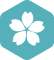### 请问Java中如何计算199阶乘

Java > Java SE [问题点数：20分，结帖人CSDN]java实现第四届蓝桥杯阶乘位数

Java计算阶乘(n!)

Java初学者作业——编写Java程序，输入一个数字，实现该数字阶乘计算

Java作业-阶乘求和

java中求5的阶乘

Java实现n阶阶乘计算

Java怎样求解n的阶乘 n!（详解）

java 之BigInteger类实现计算大整数的阶乘

package s1;import java.math.*; import java.util.*; public class A7 { public static void main(String[] args) { Scanner cin=new Scanner(System.in); int x=cin.nextInt(); BigInt

java编程题：求10的阶乘之和

* java 求10的阶乘之和 */ public class Test_10factorial { public static void main(String[] args) { int sum = 0; int num = 1; for (int i = 1; i ; i++) { num = num * i; sum += num; }

java计算问题】 1到10的阶乘，1+3+5+……+97+99的和

java求1的阶乘到10的阶乘的叠加和

public class T0302{ public static void main(String [] args){ //主方法 int a=1; //定义一个变量a int sum=0; //定义一个变量sum，把和存放在sum里 for(int i=1

java求100的阶乘

java中递归方法的应用--计算n的阶乘

java中递归方法的应用–计算n的阶乘一个方法的方法体内调用该方法本身就是递归方法。 递归要向已知的方向进行。import java.util.Scanner;public class Recursion { public static int Factorial(int n) { if (n ...

java方法计算1到20的阶乘的和

Java中求100的阶乘

java语言，用递归的方法求n的阶乘

import java.util.Scanner; //用递归的方法求n的阶乘 public class Re1 { public static void main(String args[]){ Scanner input = new Scanner(System.in); //构建一个输入实例 int n = input.nextInt(); ...

java 求1到n的阶乘

import java.util.Scanner; public class jiecheng { public static void main(String[] args) { // TODO Auto-generated method stub // int sum=0,su; // for (int i = 1; i // su=1; // for

java利用递归求5的阶乘

public class Demo2 { public static void main(String[] args) { ... * 阶乘：一个正整数的阶乘（factorial）是所有小于及等于该数的正整数的积，并且0的阶乘为1。自然数n的阶乘写作n! */ De...

java用递归方法求阶乘

java技术支持 求1~5的阶乘之和

Java中用for循环写出一个1*2*3*4*5...*n的阶乘代码实现

JAVA——递归实现n的阶乘

n的阶乘的算法：n*(n-1)(n-2)…1 例如5的阶乘为：54321 代码如下： //递归实现n的阶乘 import java.util.Scanner; public class Factorial1{ //实现阶乘的方法——使用递归 //要接收一个参数，应该定义参数...

Java-运用do-while编写10以内的阶乘求和

java递归算法求n的阶乘（n>1，n是正整数）

* 递归算法计算n的阶乘 * 递归：自己调自己 * @param n * @return */ public static Integer jieCheng(Integer n) { if (n &lt; 0) { return 0; } if (n == 1) { return 1; } return n ...

java竞赛-大数阶乘问题

Java计算阶乘（n!）需要使用实现使用BigDecimal类,因为用int最多正确算到12!,用long最多正确算到20! 计算机提供了长整型和双精度等能存储较大数的数据类型，但在有些时候，这样的数据类型不能满足实际应用的需求...

package hello;import java.util.Scanner;public class Hello {public static void main(String[] args) { Scanner in = new Scanner(System.in); int n; n=in.nextInt(); int i=1,sum=1; for(i=

Java算法 递归算法计算阶乘